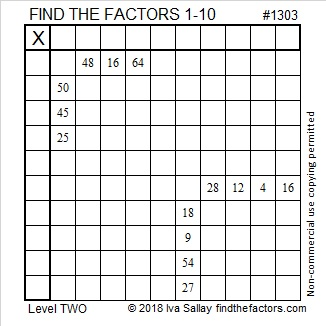# 1303 and Level 2

Multiplication tables usually have facts up to 10 × 10 = 100 or possibly 12 × 12 = 144. Numbers like 64 and 25 appear only once in those multiplication tables. Those two clues can help you get a good start solving this level 2 puzzle.Print the puzzles or type the solution in this excel file: 10-factors-1302-1310

Now I’ll share a few facts about the number 1303:

• 1303 is a prime number.
• Prime factorization: 1303 is prime.
• The exponent of prime number 1303 is 1. Adding 1 to that exponent we get (1 + 1) = 2. Therefore 1303 has exactly 2 factors.
• Factors of 1303: 1, 1303
• Factor pairs: 1303 = 1 × 1303
• 1303 has no square factors that allow its square root to be simplified. √1303 ≈ 36.09709

How do we know that 1303 is a prime number? If 1303 were not a prime number, then it would be divisible by at least one prime number less than or equal to √1303 ≈ 36.1. Since 1303 cannot be divided evenly by 2, 3, 5, 7, 11, 13, 17, 19, 23, 29 or 31, we know that 1303 is a prime number.1303 is the sum of three consecutive primes:
431 + 433 + 439 = 1303

This site uses Akismet to reduce spam. Learn how your comment data is processed.